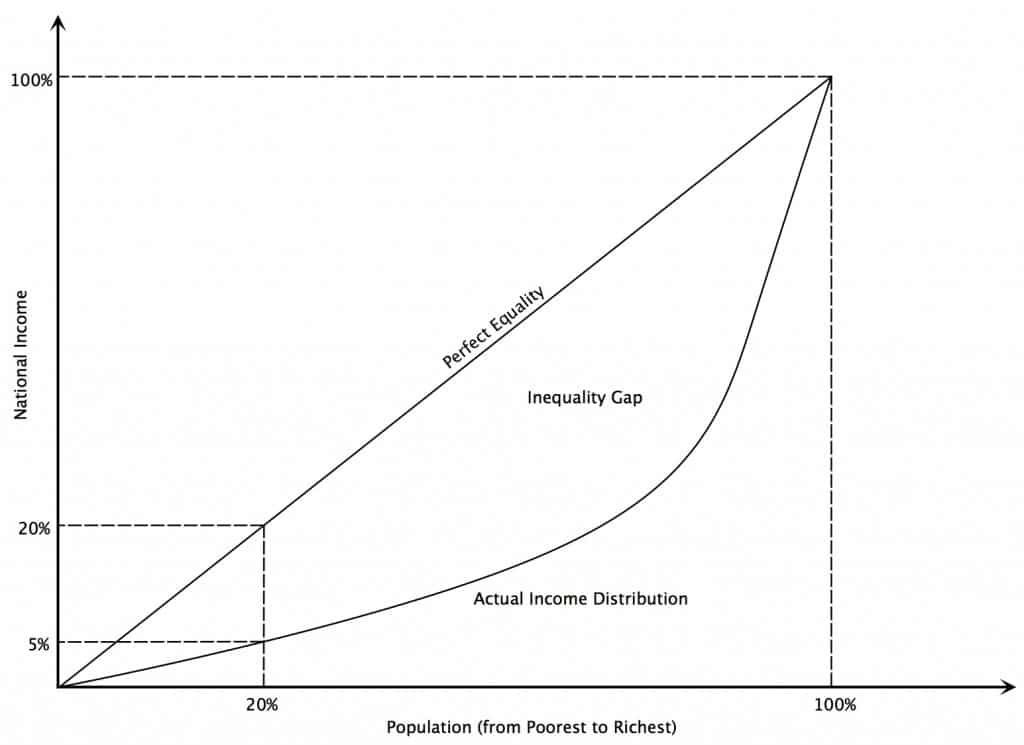# Lorenz Curve

The Lorenz curve is a graphical representation of income inequality or wealth inequality developed by American economist Max Lorenz in 1905. The graph plots percentiles of the population on the horizontal axis according to income or wealth. It plots cumulative income or wealth on the vertical axis, so that an x-value of 45 and a y-value of 14.2 would mean that the bottom 45% of the population controls 14.2% of the total income or wealth.• The Lorenz curve shows the percentage of total income earned by cumulative percentage of the population.
• In a perfectly equal society, the “poorest” 25% of the population would earn 25% of the total income, the “poorest” 50% of the population would earn 50% of the total income and the Lorenz curve would follow the path of the 45° line of equality.
• As inequality increases, the Lorenz curve deviates from the line of equality; the “poorest” 25% of the population may earn 10% of the total income; the “poorest” 50% of the population may earn 20% of the total income and so on.

The Lorenz curve is often accompanied by a straight diagonal line with a slope of 1, which represents perfect equality in income or wealth distribution; the Lorenz curve lies beneath it, showing the actual distribution. The area between the straight line and the curved line, expressed as a ratio of the area under the straight line, is the Gini coefficient, a measurement of inequality.

While the Lorenz curve is most often used to represent economic inequality, it can also demonstrate unequal distribution in any system. The farther away the curve is from the baseline, represented by the straight diagonal line, the higher the level of inequality. In economics, the Lorenz curve denotes inequality in the distribution of either wealth or income; these are not synonymous since it is possible to have high earnings but zero or negative net worth, or low earnings but a large net worth.

## One thought on “Lorenz Curve”

error: Content is protected !!